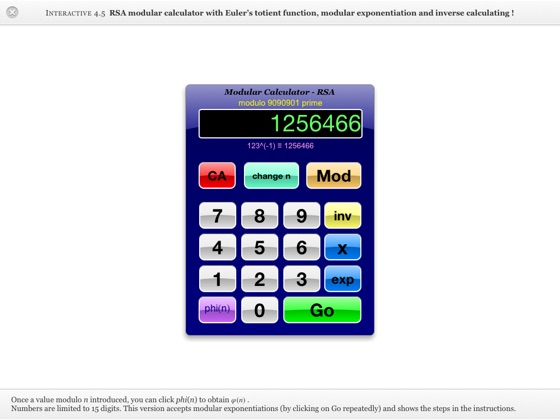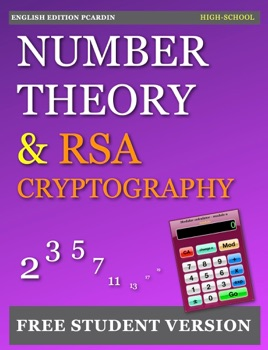## Publisher Description

This FREE STUDENT VERSION of the book contains many widgets (21) including an interactive modular calculator for RSA and interactive messages cypher / decypher (RSA 128 - 2048 bits).

In this free student version, exercices have some guidance and answers (Ch.6), but no detailed solutions (+9 Widgets Keynote see Full Version).

This book is not aimed only at students or high school teachers, but also to anyone interested in discovering the fascination of primes and understand RSA cryptography. Bases in algebra are welcome but not required.

Properties of the relation of divisibility

Fundamental theorem of arithmetic (integer factorization)

Theorem of Euclid

Fundamental theorem of primes (without proof)

Congruences and properties

Modular Arithmetic

Javascript / HTML Editor
Euclidean algorithm
Theorem GCD
Gauss Lemma
Uniqueness of the integer factorization
Modular Algebra
Chinese Remainder Theorem
Problem of Chinese cook
Euler's totient function and properties
Graph of phi(n) for n max : 50, 100, 500, 1000, 5000 and 10'000
Euler’s Theorem
Fermat’s Little theorem
A primality test
RSA Theorem (many examples)
Authentication, integrity, confidentiality, non-repudiation of data
Indicator function of Carmichael (without proof)

A final section discusses the concept of certification authority, the RSA key generation and the history of RSA (past, present and future).

A full version with detailed solutions is available on iBook Store for the price of a Caffé !

•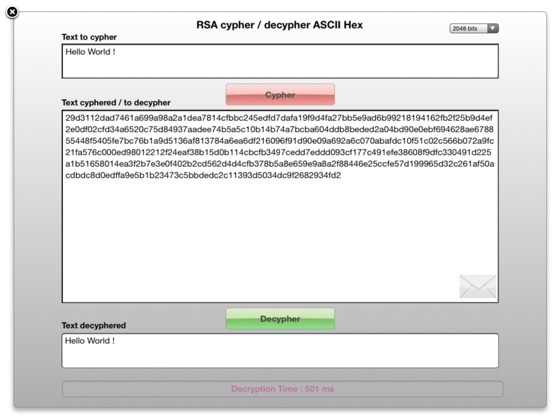•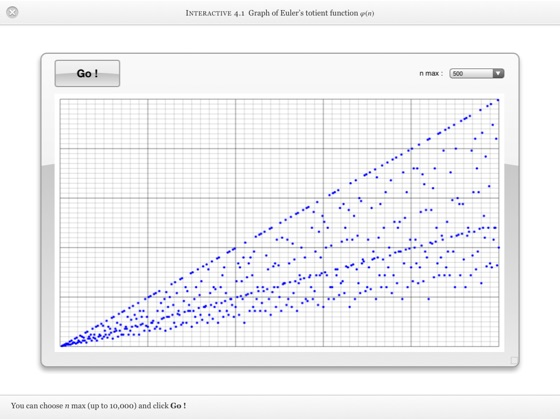••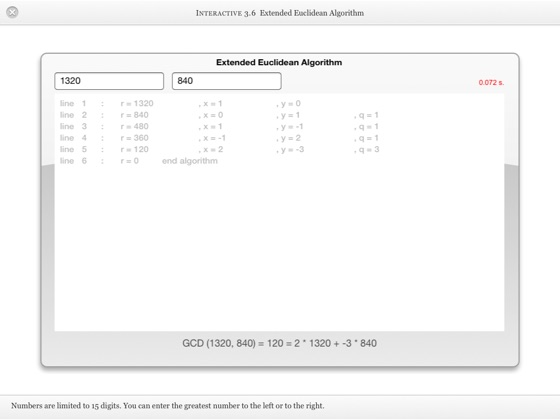•